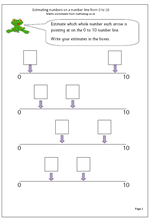# Maths worksheet: estimating on a number lineBy year 2 children should be beginning to understand the vocabulary of estimating, which includes other terms such as roughly, guess how many, about the same as, nearly, close to etc.

This worksheet looks  at estimating a whole number on a number line from 0 to 10. Probably the best technique to use is to try and estimate the middle of the line, which will be 5 and then work either from 0 to 5 or 5 to 10.

Estimating on a number line (pg 1)

## One thought on “Maths worksheet: estimating on a number line”

1. Pingback: Anonymous